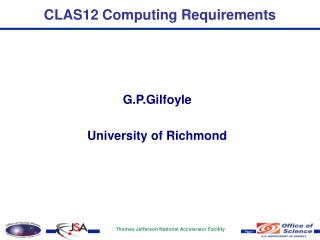DownloadDownload PresentationCLAS12 Computing Requirements

# CLAS12 Computing Requirements

Download Presentation## CLAS12 Computing Requirements

- - - - - - - - - - - - - - - - - - - - - - - - - - - E N D - - - - - - - - - - - - - - - - - - - - - - - - - - -
##### Presentation Transcript

1. CLAS12 Computing Requirements G.P.Gilfoyle University of Richmond

2. CLAS12 Computing Requirements • Assume October, 2014 start date. • Present major assumptions and results for: • Data acquisition • Calibration • Simulation • Reconstruction • Reconstruction studies • Physics analysis.

3. CLAS12 Computing Requirements Data Acquisition Assumptions: Event rate = 10 kHz Weeks running = 35 Event size = 10 kBytes 24-hour duty factor = 60% Data Rate = Event Rate x Event Size = 100 Mbyte/s Average 24-hour rate = Data Rate x 24-hour duty factor = 60 Mbyte/s Events/year = Event Rate x Weeks Running x 24-hour duty factor = 1.3x1011 Events/year Data Volume/year = Events/year x Event size = 1270 TByte/year

4. CLAS12 Computing Requirements Calibration Assumptions: CPU-time/event = 155 ms Data fraction = 5% • Data Passes = 5 Core efficiency = 90% CPU-time/year = Events/year x CPU-time/event-core x Data fraction x Data passes = 4.9x109 s Calibration Cores = (CPU-time/year)/(year in seconds x Core efficiency) = 173 cores

5. CLAS12 Computing Requirements Reconstruction - 1 Assumptions: CPU-data-time/event = 155 ms Output/input size = 2 Data passes = 2 Fraction to disk = 10% Event size = 10 kBytes Events/year = 1.3 x 1011 Data volume/year = 1.3 Pbytes/year Core efficiency = 90% CPU time/year = Data-events/year x CPU-data-time/event x Data passes = 3.9 x 1010 CPU-s/year Reconstruction Cores = CPU-time/year/(year in seconds x Core efficiency) = 1387 cores Cooked data to tape = Data volume/year x Data passes x Output/input size = 5080 TByte/year

6. CLAS12 Computing Requirements Reconstruction - 2 Assumptions CPU-data-time/event = 155 ms Output/input size = 2 and Results: Data passes = 2 Fraction to disk = 10% Event size = 10 kBytes Events/year = 1.3 x 1011 • Data volume/year = 1.3 Pbytes/year Core efficiency = 90% Disk Storage = Cooked data to tape x Fraction to disk = 508 TByte Average bandwidth = Event size x (1 + Output/input size) x Cores/(CPU-data-time/event) = 268 Mbyte/s

7. CLAS12 Computing Requirements Simulation -1 Assumptions: CPU-sim-time/event = 485 ms Events/year = 1.3 x 1011 Electron fraction = 50% Simulated/data events = 10 Analyzed fraction = 50% Multiplicity = 1.5 Core efficiency = 90% Sim-events/year = Events/year x Electron fraction x Analyzed fraction x Simulated/data events = 3.2 x 1011 CPU-sim-time/year = CPU-sim-time/event x Sim-events/year x Multiplicity = 2.3 x 1011 CPU-s/year Simulation Cores = (CPU-sim-time/year)/(year in seconds x Core efficiency) = 8,139 cores

8. CLAS12 Computing Requirements Simulation - 2 Assumptions CPU-sim-time/event = 485 ms Fraction to disk = 2% and Results: Sim-events/year = 3.2x1011 Fraction to tape = 10% Simulation Cores = 8,139 Multiplicity = 1.5 Output event size = 50 kBytes Work Disk = Sim-events/year x Output event size x Fraction to Disk = 318 TBytes Tape storage = Sim-events/year x Output event size x Fraction to Tape = 1,588 TBytes/year Average Bandwidth = (Output event size x Simulation cores)/CPU-sim-time/event = 839 MBytes/s

9. CLAS12 Computing Requirements Reconstruction Studies • Assumptions: CPU-data-time/event = 155 ms Fraction to disk = 5% Data passes = 10 Core efficiency = 90% CPU-time/year = Fraction to disk x Events/year x Data passes CPU-data-time/event = 3.4 x 1010 s Cores = CPU-time/year/(year in seconds x Core efficiency) = 1,214 cores

10. CLAS12 Computing Requirements Physics Analysis • Assumptions CPU-data-time/event-core = 8 ms Fraction of events = 50% • Data passes = 10 Core efficiency = 90% CPU-time/year = Fraction to disk x Events/year x Data passes x CPU-data-time/event = 1.7 x 1010 CPU-s/year Cores = CPU-time/year/(year in seconds x Core efficiency) = 607 cores